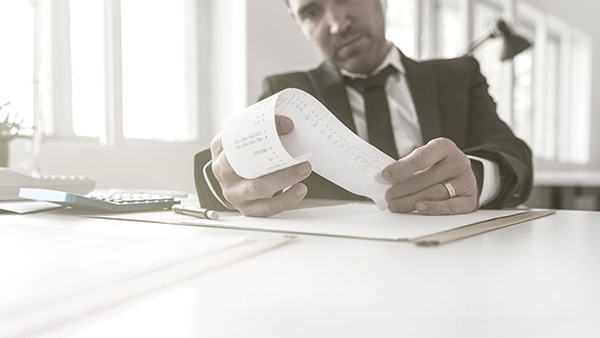10 Real Estate Calculations Every Investor Should Know | Think Realty
Article Operations

## 10 Real Estate Calculations Every Investor Should KnowUnderstand the math behind every deal.

In real estate, not everything will fit into a neat little box. Creativity is a must. However, there is indisputably a mathematical side to successful real estate. Sooner or later, you must put pen to paper and figure out if it makes sense to buy a particular property. These 10 key calculations will prove vital to your ability to analyze potential deals.

#### #1: Rent-to-Cost

Monthly Rent / Total Cost (Purchase Price + Rehab + Acquisition Costs)

Example: Rent = \$900/Month

Total Cost = \$75,000

Rent to Cost = 1.2% (\$900 / \$75,000)

When to use it: If you need to quickly determine if a potential rental is worth evaluating further.

What you need to know:

• Cheaper properties have higher rent-to-cost
• Use this to compare properties, not make a final decision
• Buy-and-hold investors should look for a value greater than 1 percent

#### #2: Gross Yield

Annual Rent / Total Cost

Example: Rent = \$900/Month, \$10,800/Year

Total Cost = \$75,000.

Gross Yield = 14.4% Gross Yield (\$10,800 / \$75,000)

When to use it: In context with institutional investors. Gross yield uses the same values as rent-to-cost.

#### #3: Gross Rent Calculator

Sale Price / Gross Annual Rents

Example: Sale Price = \$500,000

Annual Rents = \$50,000

Gross Rent Multiplier = 10 (\$500,000 / \$50,000)

When to use it: When looking for a quick sign a potential commercial deal (multifamily or otherwise) is worth considering.

What you need to know:

• Does not take operating expenses into account
• May be calculated using sales price or total cost (including rehab and acquisition)

#### #4: Cap Rate

(Net Operating Income / Total Cost of Property) x 100

Example: Net Operating Income (NOI) = \$80,000

Total Cost = \$1,000,000

Cap Rate = 8.0 (\$80,000 / \$1,000,000) x 100

When to use it: When you need a way to compare the returns on different investment properties.

What you need to know:

• Cap rate does not include debt servicing costs
• Can be calculated using different definitions of cost and NOI
• Best used when derived using actual income and expense numbers from the previous year
• Beware of pro forma numbers

#### #5: Debt Service Coverage Rate (DSCR)

Net Operating Income / Annual Debt Service

Example: NOI = \$120,000

Annual Debt Service = \$100,000

DSCR = 1.2 (\$120,000 / \$100,000)

When to use it: To evaluate the viability of an investment from a lending perspective.

What you need to know:

• Banks use DSCR to predict whether a borrower is likely to repay a loan.
• Compares an investment’s income to the amount of the loan payment (interest and principal)
• Most bank lenders want DSCR to be at least 1.2

#### #6: The 50% Rule

Gross Rents x 0.5 = Approximate Annual Operating Expenses

Example: Gross Rents = \$200,000

50% Rule: \$200,000 X 0.5 = \$100,000 (Approximate Annual Operating Expenses)

When to use it: When you need a fast estimate for operating expenses.

What you need to know:

• Usually used with apartments
• Can be applied using real numbers or approximations
• May be inserted into cap rate and DSCR estimates

#### #7: The 70% Rule

Strike Price = (0.7 x After Repair Value) – Rehab

Example: After Repair Value (ARV) = \$200,000

Rehab = \$30,000

Strike Price =  (0.7 x 200,000) – \$30,000 =\$110,000

When to use it: To determine the strike price most optimal for solid profits on a potential investment.

What you need to know:

• Usually used for flips
• The best ARV values are derived using comparable costs from comparable properties
• Finalize your strike price after a detailed analysis that includes carrying costs

#### #8: Cash on Cash Return

(Net Operating Income – Annual Debt Service) / Cash in Property

Example: NOI = \$120,000

Annual Debt Service = \$100,000

Cash in Property = \$100,000

Cash on Cash Return =  (\$120,000 – \$100,000) / \$100,000 = 20%

When to use it: To evaluate the success of an investment after its first year.

What you need to know:

• This only provides a return value for one year

#### #9: Return on Investment (ROI)

(Gain on Investment – Cost of Investment) / Cost of Investment

Example: Gain on Investment = \$20,000

Cost of Investment = \$50,000. Years owned = 5

ROI = 40% (\$20,000 / \$50,000)

Annualized Return (AR): ROI/# years you owned the property

AR = 8% (40%/5)

When to use it: To get a “whole picture” of a future investment or a completed investment.

What you need to know:

• Use ROI to calculate annualized returns by dividing ROI by the number of years you have owned the property.

#### #10: Internal Rate of Return (IRR)

IRR is defined as “the discount rate that makes an investment’s net present value equal to zero.” IRR accounts for when you spend and receive cash. For most investors, it is better to receive cash sooner rather than later because then you can reinvest it. You will need an IRR calculator (my favorite is the IRR function available in Excel) to derive it.

When to use it: To put your ROI in perspective with your initial outlay of money or compare investment opportunities in different asset classes.

What you need to know:

• IRR may also be referred to as “economic rate of return” or “discounted cash flow rate of return”
• IRR omits external factors like cost of capital and inflation
• In most cases, the higher a project’s internal rate of return, the more desirable it is

Category: Article, Operations
RECENT ARTICLES All Articles